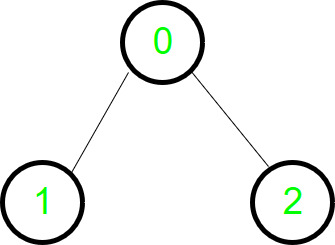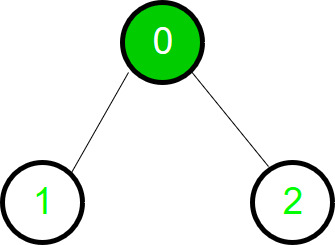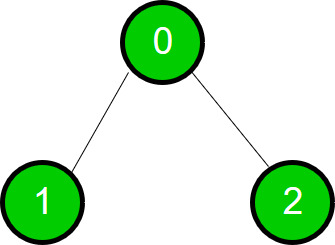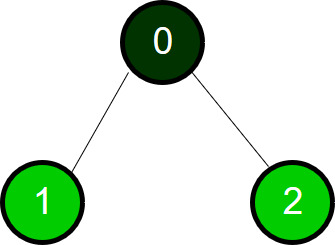# BFS using vectors & queue as per the algorithm of CLRS

Breadth-first search traversal of a graph using the algorithm given in CLRS book.

BFS is one of the ways to traverse a graph. It is named so because it expands the frontier between discovered and undiscovered vertices uniformly across the breadth of the frontier. What it means is that the algorithm first discovers all the vertices connected to “u” at a distance of k before discovering the vertices at a distance of k+1 from u. The algorithm given in CLRS uses the concept of “colour” to check if a vertex is discovered fully or partially or undiscovered. It also keeps a track of the distance a vertex u is from the source s.

```BFS(G,s)
1  for each vertex u in G.V - {s}
2     u.color = white
3     u.d = INF
4     u.p = NIL
5  s.color = green
6  s.d = 0
7  s.p = NIL
8  Q = NULL
9  ENQUEUE(Q,s)
10 while Q != NULL
11    u = DEQUEUE(Q)
12    for each v in G.Adj[u]
13       if v.color == white
14          v.color = green
15          v.d = u.d + 1
16          v.p = u
17          ENQUEUE(Q,v)
18    u.color = dark_green
```

It produces a “breadth-first tree” with root s that contains all reachable vertices. Let’s take a simple directed graph and see how BFS traverses it.The graphStarting of traversal1st traversal1st traversal completes

## C++

 `// CPP program to implement BFS as per CLRS  ` `// algorithm. ` `#include ` `using` `namespace` `std; ` ` `  `// Declaring the vectors to store color, distance ` `// and parent ` `vector colour; ` `vector<``int``> d; ` `vector<``int``> p; ` ` `  `/* This function adds an edge to the graph. ` `It is an undirected graph. So edges are  ` `added for both the nodes. */` `void` `addEdge(vector <``int``> g[], ``int` `u, ``int` `v) ` `{ ` `    ``g[u].push_back(v); ` `    ``g[v].push_back(u); ` `} ` ` `  `/* This function does the Breadth First Search*/` `void` `BFSSingleSource(vector <``int``> g[], ``int` `s) ` `{ ` `    ``// The Queue used for the BFS operation ` `    ``queue<``int``> q; ` ` `  `    ``// Pushing the root node inside the queue ` `    ``q.push(s);  ` ` `  `    ``/* Distance of root node is 0 & colour ` `    ``is gray as it is visited partially now */` `    ``d[s] = 0; ` `    ``colour[s] = ``"green"``; ` `         `  `    ``/* Loop to traverse the graph. Traversal ` `    ``will happen traverse until the queue is  ` `    ``not empty.*/` `    ``while` `(!q.empty()) ` `    ``{ ` `        ``/* Extracting the front element(node)  ` `        ``and poping it out of queue. */` `        ``int` `u = q.front(); ` `        ``q.pop(); ` ` `  `        ``cout << u << ``" "``; ` ` `  `        ``/* This loop traverses all the child nodes of u */` `        ``for` `(``auto` `i = g[u].begin(); i != g[u].end(); i++) ` `        ``{ ` `            ``/* If the colour is white then the said node ` `            ``is not traversed. */` `            ``if` `(colour[*i] == ``"white"``) ` `            ``{ ` `                ``colour[*i] = ``"green"``; ` `                ``d[*i] = d[u] + 1; ` `                ``p[*i] = u; ` ` `  `                ``/* Pushing the node inside queue ` `                ``to traverse its children. */` `                ``q.push(*i);  ` `            ``} ` `        ``} ` ` `  `        ``/* Now the node u is completely traversed ` `        ``and colour is changed to black. */` `        ``colour[u] = ``"dark_green"``; ` `    ``} ` `} ` ` `  `void` `BFSFull(vector <``int``> g[], ``int` `n) ` `{ ` `    ``/* Initially all nodes are not traversed. ` `    ``Therefore, the colour is white. */` `    ``colour.assign(n, ``"white"``); ` `    ``d.assign(n, 0); ` `    ``p.assign(n, -1); ` ` `  `    ``// Calling BFSSingleSource() for all white ` `    ``// vertices. ` `    ``for` `(``int` `i = 0; i < n; i++)      ` `        ``if` `(colour[i] == ``"white"``) ` `            ``BFSSingleSource(g, i);  ` `} ` ` `  `// Driver Function ` `int` `main() ` `{ ` `    ``// Graph with 7 nodes and 6 edges. ` `    ``int` `n = 7; ` `         `  `    ``// The Graph vector ` `    ``vector <``int``> g[n]; ` `     `  `    ``addEdge(g, 0, 1); ` `    ``addEdge(g, 0, 2); ` `    ``addEdge(g, 1, 3); ` `    ``addEdge(g, 1, 4); ` `    ``addEdge(g, 2, 5); ` `    ``addEdge(g, 2, 6); ` ` `  `    ``BFSFull(g, n); ` ` `  `    ``return` `0; ` `} `

## Python3

 `# Python3 program to implement BFS as  ` `# per CLRS algorithm.  ` `import` `queue  ` ` `  `# This function adds an edge to the graph.  ` `# It is an undirected graph. So edges  ` `# are added for both the nodes.  ` `def` `addEdge(g, u, v): ` `    ``g[u].append(v)  ` `    ``g[v].append(u) ` ` `  `# This function does the Breadth ` `# First Search ` `def` `BFSSingleSource(g, s): ` `     `  `    ``# The Queue used for the BFS operation  ` `    ``q ``=` `queue.Queue() ` ` `  `    ``# Pushing the root node inside  ` `    ``# the queue  ` `    ``q.put(s)  ` ` `  `    ``# Distance of root node is 0 & colour is   ` `    ``# gray as it is visited partially now  ` `    ``d[s] ``=` `0` `    ``colour[s] ``=` `"green"` `         `  `    ``# Loop to traverse the graph. Traversal  ` `    ``# will happen traverse until the queue  ` `    ``# is not empty. ` `    ``while` `(``not` `q.empty()): ` `         `  `        ``# Extracting the front element(node)  ` `        ``# and poping it out of queue.  ` `        ``u ``=` `q.get()  ` ` `  `        ``print``(u, end ``=` `" "``) ` ` `  `        ``# This loop traverses all the child ` `        ``# nodes of u ` `        ``i ``=` `0` `        ``while` `i < ``len``(g[u]): ` `             `  `            ``# If the colour is white then  ` `            ``# the said node is not traversed.  ` `            ``if` `(colour[g[u][i]] ``=``=` `"white"``): ` `                ``colour[g[u][i]] ``=` `"green"` `                ``d[g[u][i]] ``=` `d[u] ``+` `1` `                ``p[g[u][i]] ``=` `u  ` ` `  `                ``# Pushing the node inside queue  ` `                ``# to traverse its children.  ` `                ``q.put(g[u][i]) ` `            ``i ``+``=` `1` ` `  `        ``# Now the node u is completely traversed  ` `        ``# and colour is changed to black.  ` `        ``colour[u] ``=` `"dark_green"` ` `  `def` `BFSFull(g, n): ` `     `  `    ``# Initially all nodes are not traversed.  ` `    ``# Therefore, the colour is white.  ` `    ``colour ``=` `[``"white"``] ``*` `n  ` `    ``d ``=` `[``0``] ``*` `n  ` `    ``p ``=` `[``-``1``] ``*` `n  ` ` `  `    ``# Calling BFSSingleSource() for all  ` `    ``# white vertices ` `    ``for` `i ``in` `range``(n): ` `        ``if` `(colour[i] ``=``=` `"white"``):  ` `            ``BFSSingleSource(g, i) ` ` `  `# Driver Code ` ` `  `# Graph with 7 nodes and 6 edges.  ` `n ``=` `7` ` `  `# Declaring the vectors to store color,  ` `# distance and parent  ` `colour ``=` `[``None``] ``*` `n ` `d ``=` `[``None``] ``*` `n ` `p ``=` `[``None``] ``*` `n ` `     `  `# The Graph vector  ` `g ``=` `[[] ``for` `i ``in` `range``(n)]  ` ` `  `addEdge(g, ``0``, ``1``)  ` `addEdge(g, ``0``, ``2``)  ` `addEdge(g, ``1``, ``3``)  ` `addEdge(g, ``1``, ``4``)  ` `addEdge(g, ``2``, ``5``)  ` `addEdge(g, ``2``, ``6``)  ` ` `  `BFSFull(g, n) ` ` `  `# This code is contributed by Pranchalk `

Output:

```0 1 2 3 4 5 6
```

Attention reader! Don’t stop learning now. Get hold of all the important DSA concepts with the DSA Self Paced Course at a student-friendly price and become industry ready.

My Personal Notes arrow_drop_upCheck out this Author's contributed articles.

If you like GeeksforGeeks and would like to contribute, you can also write an article using contribute.geeksforgeeks.org or mail your article to contribute@geeksforgeeks.org. See your article appearing on the GeeksforGeeks main page and help other Geeks.

Please Improve this article if you find anything incorrect by clicking on the "Improve Article" button below.

Article Tags :
Practice Tags :

4

Please write to us at contribute@geeksforgeeks.org to report any issue with the above content.